# Maths in IT #2: Venn diagrams

Hey everyone, welcome back to part two of the Maths in IT series. I got a lot of positive response, so I guess I should just keep doing what I was already doing. This post will continue where part one left off, so if you haven’t read it I suggest you do so now before continuing.

1. Maths in IT #1: Basic set theory
2. Maths in IT #2: Venn diagrams
3. Maths in IT #3: Algebra of sets
4. Coming soon…

Here’s a quick cheat sheet with symbols I’ll use in this article:
Explicit definition:$A = \{a, b, c\}$
Implicit definition:$A = \{x | \text{ x is a letter in the alphabet}\}$
a is an element of A:$a \in A$
a is not an element of A:$a \notin A$
A is a subset of B:$A \subset B$
Empty set:$\emptyset$

## Venn diagrams

As promised we’ll use this post to combine sets. Before we do that let’s take a look at how to visually represent collection. We can do this using a Venn diagram. A Venn diagram is about as simple as it gets (although they can be pretty complex too). Each set is represented by a circle. The diagram can illustrate relationships between the represented sets.

Let’s look at an example. We have two collections,$A = \{a, b, c\}$ and$B = \{d, e, f\}$. Let’s show them in a Venn diagram.A Venn diagram

When two sets have no shared elements, or for every$x \in A$ goes that$x \notin B$, we say the sets are disjoint.

Now suppose$A \subset B$ (A is a subset of B). We can show this in a Venn diagram and you’ll recognize it immediately.A Venn diagram of a subset

When A is a subset of B then B overlaps A completely.

In the next sections we’ll combine sets and use Venn diagrams to visualize what elements we’re interested in.

## Intersection

So suppose we have two collections,$A = \{a, b, c, d\}$ and$B = \{c, d, e, f\}$. If I asked you which elements are in both A and B you’d answer c and d.

This is called the intersection of A and B.
We can write this as$A \cap B$.
In this example we can say$A \cap B = \{c, d\}$
More formally we say that$A \cap B = \{x | x \in A \text{ and } x \in B\}$.
And the symbol for “and” is actually$\land$, so to formalize it completely:$A \cap B = \{x | x \in A \land x \in B\}$

Phew, that looks a whole lot like maths! You should read that as “the intersection of A and B is the collection of every x where x is an element of A and x is an element of B.”
And here is the Venn diagram, which visualizes this nicely. The intersection is the part in the thick black line where A and B overlap.A Venn diagram of an intersection

With an intersection we can give a formal definition of disjoint sets. When$A \cap B = \emptyset$ then A and B are disjoint.

Furthermore we can say that for any collection A goes that$A \cap \emptyset = \emptyset$.
Also$A \cap A = A$.
And when$A \subset B$ then$A \cap B = A$ (check the subset Venn diagram).

## Union

The intersection of two sets is the set of elements x where x is in A and B. Likewise, the union of two sets is the set of elements that are in set A or B. So when we have$A = \{a, b, c, d\}$ and$B = \{c, d, e, f\}$ then the union of A and B is$\{a, b, c, d, e, f\}$ (no doubles).

We can write a union of A and B as$A \cup B$.
Like$\land$ is the symbol for “and”$\lor$ is the symbol for “or”. So the formal definition of union is as follows:$A \cup B = \{x | x \in A \lor x \in B\}$

Read that as “the union of A and B is the collection of every x where x is an element of A or x is an element of B.”
In a Venn diagram the union is basically just both sets (the part in the thick black line).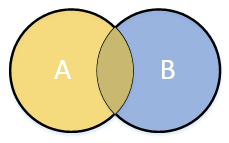A Venn diagram of an overlapping union

Unlike an intersection, a union of disjoint sets is not an empty set (notice that both sets have a thick black line).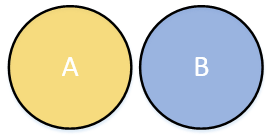A Venn diagram of a union

Now for any collection A goes that$A \cup \emptyset = A$.
And, again,$A \cup A = A$.
Also when$A \subset B$ then$A \cup B = B$ (check the subset Venn diagram).

## Universe and Complement

When we’re talking about sets we’re usually not talking about that set in isolation. When I tell you that non-smokers live longer you understand that they live longer compared to people that do smoke. And you also understand that non-smokers and smokers combined make up for the worlds population. In this case we’re saying that we have a set of non-smokers in a universe of all people. We denote a universe with the capital letter U.

For every set$A_1, A_2, A_3... A_n$ goes that$A_n \subset U$.
That makes sense as U represents all elements we wish to consider for our case. No collection can ever contain an element that is not a part of all elements.

In a Venn diagram we draw a universe as a rectangle in which all our sets are drawn. In the following example N is the set of non-smokers and U is the universe containing all people.A Venn diagram of a universe

Now with the notion of a universe we can say we want all elements that are not in any collection A. We call this the complement of A and we write it as$A^c$. In the following Venn diagram the white part represents$A^c$.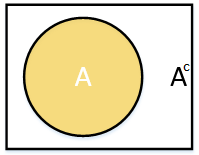A Venn diagram of a complement

We can now formally define the complement:$A^c = \{ x | x \in U \land x \notin A \}$

It goes without saying, but$\emptyset^c = U$ and$U^c = \emptyset$.
A little less obvious is that$(A^c)^c = A$. It makes sense though, as we first take everything that isn’t A (the complement of A) and then we take everything that isn’t in the resulting set, but that is A. Try drawing it in a Venn diagram and you’ll see what I mean.

A complement relative to a universe is called an absolute complement. A complement can also be relative to other sets. For example, when no universe is defined and we have sets A and B then the relative complement of A in B is the set of elements of B that are not in A. This takes the form of$B \cap A^c$ or$A \setminus B$.$A \setminus B = \{ x | x \in B \land x \notin A \}$

In a Venn diagram$A \setminus B$ look as follows:## Combining sets

We can now combine sets using intersection, union and complements. For example, let’s say our universe U is all living creatures on earth. Within U we have sets M, containing all mammals, B, containing all birds, and E, containing all animals that lay eggs. Formally:$U = \{ x | \text{x is an animal} \}$$M = \{ x | x \in U \land \text{x is a mammal} \}$$B = \{ x | x \in U \land \text{x is a bird} \}$$E = \{ x | x \in U \land \text{x lays eggs} \}$
Giving the following Venn diagram we can already draw some conclusions.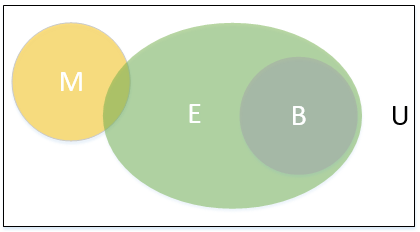A Venn diagram with multiple sets

We can see that all birds lay eggs. Some mammals lay eggs too. No animal is both a bird and a mammal. As you see Venn diagrams can be really useful.
Now suppose we want the set of all mammals that lay eggs and also all birds. This is the collection$(M \cap E) \cup B$. That is, we take the intersection of M and E and union the result with B. In a Venn diagram we can see this collection (the red colored parts).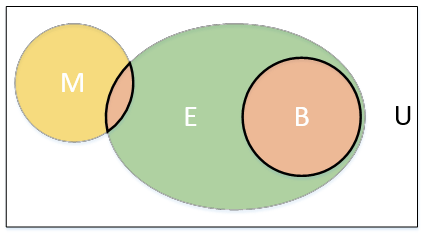A Venn diagram with multiple sets

Now we can get every combination of sets using intersection, union and complement. It’s not always easy, but it’s possible.

## Some code

As promised I’m going to keep things practical. So what’s the practical use of all this? Well, most languages let you work with exactly these functions!

For example, take a look at this SQL expression.

(SELECT 1
UNION SELECT 2
UNION SELECT 3)
INTERSECT
(SELECT 3
UNION SELECT 4)

What will that return? It returns only 3 because 3 is an element of both collections. This example also shows the UNION operator. Basically this is the set$(\{1\} \cup \{2\} \cup \{3\}) \cap (\{3\} \cup \{4\})$.

And SQL knows complement too.

(SELECT 1
UNION SELECT 2
UNION SELECT 3)
EXCEPT
(SELECT 3
UNION SELECT 4)

What happens here is that we have (an implied) universe$U = \{1, 2, 3, 4\}$ and EXCEPT is the complement. So this query is basically the formula$\{3, 4\}^c$.
We could also say that U is not defined and this is the relative complement of$\{3, 4\}$ in$\{1, 2, 3\}$:$\{3, 4\} \setminus \{1, 2, 3\} = \{1, 2\}$.

But what about C#? In my previous post we’ve seen HashSet<T>, but I’m not going to use that now. Instead I’m just going with a List<T> as LINQ provides various extension methods for working with sets. Notice that HashSet<T> has its own methods in addition to the LINQ methods.

List<int> a = new List<int>();
a.Add(1);
a.Add(2);
a.Add(3);

List<int> b = new List<int>();
b.Add(3);
b.Add(4);

List<int> intersect = a.Intersect(b).ToList();
List<int> union = a.Union(b).ToList();
List<int> except = a.Except(b).ToList();

You may wonder what that looks like in Haskell (since I’ve shown you Haskell the last time too), but it’s not really that different.

Prelude> import Data.List
Prelude Data.List> [1, 2, 3] intersect [3, 4]

Prelude Data.List> [1, 2, 3] union [3, 4]
[1,2,3,4]
Prelude Data.List> [1, 2, 3] \\ [3, 4]
[1,2]

I’ve actually needed this stuff in my day to day work. It’s not that hard and sometimes it’s an explicit business requirement. For example I needed all sales orders from The Netherlands, Belgium and Luxembourg (the BeNeLux). That’s really just a union! And I’ve needed intersect too, give me all Dutch customers that are not in some list of customers. And how about all non-Dutch customers? That’s just the complement!

Next time I’d like to continue with algebra and algebra of sets in specific. Does that sound like it will give you nightmares? Don’t worry, I’ll be gentle!

See you next time!